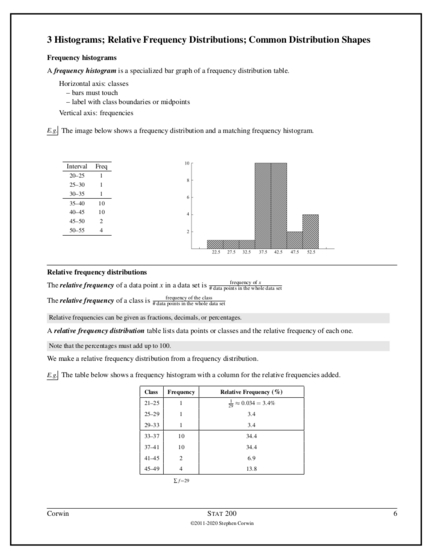# Relative Frequency Distributions and HistogramsContributed by:This pdf includes the following topics:-
Frequency histograms
Relative frequency distributions
Relative frequency histograms
Common distribution shapes
1. 3 Histograms; Relative Frequency Distributions; Common Distribution Shapes
Frequency histograms
A frequency histogram is a specialized bar graph of a frequency distribution table.
Horizontal axis: classes
– bars must touch
– label with class boundaries or midpoints
Vertical axis: frequencies
E.g. The image below shows a frequency distribution and a matching frequency histogram.
Interval Freq
20–25 1
25–30 1
30–35 1
35–40 10
40–45 10
45–50 2
50–55 4
Relative frequency distributions
frequency of x
The relative frequency of a data point x in a data set is # data points in the whole data set
frequency of the class
The relative frequency of a class is # data points in the whole data set
Relative frequencies can be given as fractions, decimals, or percentages.
A relative frequency distribution table lists data points or classes and the relative frequency of each one.
Note that the percentages must add up to 100.
We make a relative frequency distribution from a frequency distribution.
E.g. The table below shows a frequency histogram with a column for the relative frequencies added.
Class Frequency Relative Frequency (%)
1
21–25 1 29 ≈ 0.034 = 3.4%
25–29 1 3.4
29–33 1 3.4
33–37 10 34.4
37–41 10 34.4
41–45 2 6.9
45–49 4 13.8
∑ f =29
Corwin S TAT 200 6
2. Relative frequency histograms
A relative frequency histogram is a histogram for a relative frequency distribution.
E.g. The relative frequency histogram shown below matches the frequency distribution shown.
Class Frequency Relative
Frequency (%)
20–25 1 3.4
25–30 1 3.4
30–35 1 3.4
35–40 10 34.5
40–45 10 34.5
45–50 2 6.9
50–55 4 14.0
Σ f = 29
The Greek capital letter sigma (Σ) means “add up the values” (i.e., “sum”). Notice that the sum of the frequencies is the
total number of data points.
Corwin S TAT 200 7
3. E.g. Consider the following data set.
44 39 42 37 39 40 37 36 38 43
41 43 37 36 41 39 40 38 40 43
(a) Construct a frequency distribution for the data set using the classes 34–37, 37–40, 40–43, 43–46.
Class Frequency
34–37 2
37–40 8
40–43 6
43–46 4
(b) Construct a frequency histogram for the data set.
Class Frequency Rel Freq (%)
2
34–37 2 20 = 0.1
37–40 8 0.4
40–43 6 0.3
43–46 4 0.2
(d) Construct a relative frequency histogram for the data set.
Corwin S TAT 200 8